0
In cart:
0 items\$0.00
Search and Filter Items
Search Products
OR Browse Departments

Filter Results

# Hard and Semi Hard Cheese

Product Size Price Quantity

## Beemster XO Gouda

Per Pound
\$21.99
lb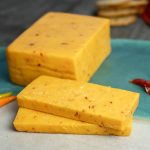CFL
Per Pound
\$5.99
lb

CFL
Per Pound
\$5.99
lb

CFL
Per Pound
\$6.99
lb

CFL
Per Pound
\$16.99
lb

CFL
Per Pound
\$14.99
lb

## Caves of Faribault Jeff’s Select Gouda

CFL
Per Pound
\$20.99
lb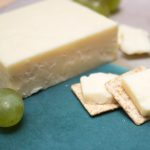## Cow Caviar Sharp Cheddar Cheese

CFL
Each (price approx.)
\$4.47You are ordering units, but this item is sold by the pound. The price per pound is \$7.99 and the average weight for one item is 0.56 lbs., therefore, the approximate price for one item is \$4.47. Please order the number of items you would like to receive. Your final price will be calculated based on the total weight of the actual items fulfilled.

## Edelweiss Gouda Cheese

CFL
Per Pound
\$14.99
lb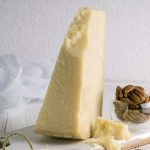## Italy Parmigiano Reggiano Cheese

Each (price approx.)
\$5.00You are ordering units, but this item is sold by the pound. The price per pound is \$19.99 and the average weight for one item is 0.25 lbs., therefore, the approximate price for one item is \$5.00. Please order the number of items you would like to receive. Your final price will be calculated based on the total weight of the actual items fulfilled.

## Italy Pecorino Romano Cheese

Each (price approx.)
\$3.90You are ordering units, but this item is sold by the pound. The price per pound is \$14.99 and the average weight for one item is 0.26 lbs., therefore, the approximate price for one item is \$3.90. Please order the number of items you would like to receive. Your final price will be calculated based on the total weight of the actual items fulfilled.

## Kerrygold Dubliner Irish Aged Cheddar Cheese

Each (price approx.)
\$6.84You are ordering units, but this item is sold by the pound. The price per pound is \$17.99 and the average weight for one item is 0.38 lbs., therefore, the approximate price for one item is \$6.84. Please order the number of items you would like to receive. Your final price will be calculated based on the total weight of the actual items fulfilled.

CFL
Per Pound
\$9.99
lb

CFL
Per Pound
\$19.99
lb

## Milton Creamery Caramelized Onion Cheddar Cheese

CFL
Per Pound
\$11.99
lb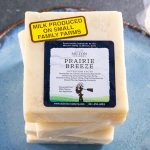## Milton Creamery Prairie Breeze Cheese

CFL
Each (price approx.)
\$5.25You are ordering units, but this item is sold by the pound. The price per pound is \$14.99 and the average weight for one item is 0.35 lbs., therefore, the approximate price for one item is \$5.25. Please order the number of items you would like to receive. Your final price will be calculated based on the total weight of the actual items fulfilled.

## Murray Australian Cheddar Cheese

Each (price approx.)
\$3.96You are ordering units, but this item is sold by the pound. The price per pound is \$8.99 and the average weight for one item is 0.44 lbs., therefore, the approximate price for one item is \$3.96. Please order the number of items you would like to receive. Your final price will be calculated based on the total weight of the actual items fulfilled.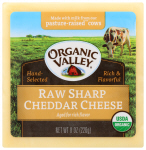## Organic Valley Organic Raw Sharp Cheddar Cheese

CFLOG
8 oz
\$7.99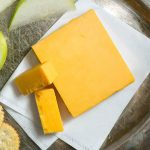## Pasture Pride Cheddar Cheese

CFL
Each (price approx.)
\$4.28You are ordering units, but this item is sold by the pound. The price per pound is \$6.49 and the average weight for one item is 0.66 lbs., therefore, the approximate price for one item is \$4.28. Please order the number of items you would like to receive. Your final price will be calculated based on the total weight of the actual items fulfilled.

Per Pound
\$8.99
lb

CFL
0.833 oz
\$0.99

Per Pound
\$5.49
lb

CF
Per Pound
\$11.99
lb

CF
Per Pound
\$7.99
lb

## Sartori Bellavitano Gold Cheese

CFL
Per Pound
\$16.99
lb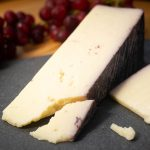CFL
Per Pound
\$16.99
lb

## Sartori Montamore Cheese

CFL
Each (price approx.)
\$9.43You are ordering units, but this item is sold by the pound. The price per pound is \$15.99 and the average weight for one item is 0.59 lbs., therefore, the approximate price for one item is \$9.43. Please order the number of items you would like to receive. Your final price will be calculated based on the total weight of the actual items fulfilled.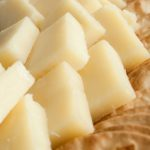## Sartori Parmesan Cheese

CFL
Each (price approx.)
\$7.13You are ordering units, but this item is sold by the pound. The price per pound is \$13.99 and the average weight for one item is 0.51 lbs., therefore, the approximate price for one item is \$7.13. Please order the number of items you would like to receive. Your final price will be calculated based on the total weight of the actual items fulfilled.

CFL
Per Pound
\$16.99
lb

## Sartori Wisantigo Asiago Cheese

CFL
Per Pound
\$11.49
lb## Swiss Valley Swiss Cheese

CFL
Each (price approx.)
\$4.00You are ordering units, but this item is sold by the pound. The price per pound is \$7.99 and the average weight for one item is 0.50 lbs., therefore, the approximate price for one item is \$4.00. Please order the number of items you would like to receive. Your final price will be calculated based on the total weight of the actual items fulfilled.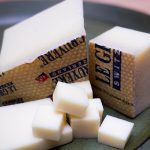## Switzerland Swiss Gruyere Cheese

Each (price approx.)
\$5.60You are ordering units, but this item is sold by the pound. The price per pound is \$19.99 and the average weight for one item is 0.28 lbs., therefore, the approximate price for one item is \$5.60. Please order the number of items you would like to receive. Your final price will be calculated based on the total weight of the actual items fulfilled.Per Pound
\$19.99
lb

## Tillamook 2 Year Sharp Cheddar Cheese

Each (price approx.)
\$4.56You are ordering units, but this item is sold by the pound. The price per pound is \$11.99 and the average weight for one item is 0.38 lbs., therefore, the approximate price for one item is \$4.56. Please order the number of items you would like to receive. Your final price will be calculated based on the total weight of the actual items fulfilled.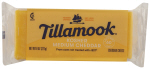8 oz
\$5.99

## Tillamook White Cheddar Cheese

Each (price approx.)
\$4.42You are ordering units, but this item is sold by the pound. The price per pound is \$12.99 and the average weight for one item is 0.34 lbs., therefore, the approximate price for one item is \$4.42. Please order the number of items you would like to receive. Your final price will be calculated based on the total weight of the actual items fulfilled.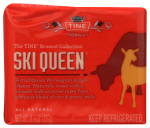8.8 oz
\$9.99

## Wales Red Dragon Cheddar Cheese

Per Pound
\$22.99
lb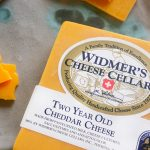CFL
Per Pound
\$12.99
lb

CFL
Per Pound
\$18.99
lb